• DeepLearning tutorial（3）MLP多层感知机原理简介+代码详解 @author：wepon @blog：http://blog.csdn.net/u012162613/article/details/43221829 本文介绍多层感知机算法，特别是详细解读其代码实现，基于...
DeepLearning tutorial（3）MLP多层感知机原理简介+代码详解

@author：wepon

@blog：http://blog.csdn.net/u012162613/article/details/43221829

本文介绍多层感知机算法，特别是详细解读其代码实现，基于python theano，代码来自：Multilayer Perceptron，如果你想详细了解多层感知机算法，可以参考：UFLDL教程，或者参考本文第一部分的算法简介。

经详细注释的代码：放在我的github地址上，可下载。

一、多层感知机（MLP）原理简介

多层感知机（MLP，Multilayer Perceptron）也叫人工神经网络（ANN，Artificial Neural Network），除了输入输出层，它中间可以有多个隐层，最简单的MLP只含一个隐层，即三层的结构，如下图：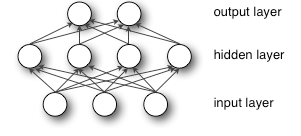从上图可以看到，多层感知机层与层之间是全连接的（全连接的意思就是：上一层的任何一个神经元与下一层的所有神经元都有连接）。多层感知机最底层是输入层，中间是隐藏层，最后是输出层。

输入层没什么好说，你输入什么就是什么，比如输入是一个n维向量，就有n个神经元。

隐藏层的神经元怎么得来？首先它与输入层是全连接的，假设输入层用向量X表示，则隐藏层的输出就是

f(W1X+b1)，W1是权重（也叫连接系数），b1是偏置，函数f 可以是常用的sigmoid函数或者tanh函数：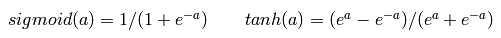最后就是输出层，输出层与隐藏层是什么关系？其实隐藏层到输出层可以看成是一个多类别的逻辑回归，也即softmax回归，所以输出层的输出就是softmax(W2X1+b2)，X1表示隐藏层的输出f(W1X+b1)。

MLP整个模型就是这样子的，上面说的这个三层的MLP用公式总结起来就是，函数G是softmax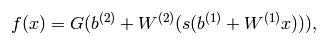因此，MLP所有的参数就是各个层之间的连接权重以及偏置，包括W1、b1、W2、b2。对于一个具体的问题，怎么确定这些参数？求解最佳的参数是一个最优化问题，解决最优化问题，最简单的就是梯度下降法了（SGD）：首先随机初始化所有参数，然后迭代地训练，不断地计算梯度和更新参数，直到满足某个条件为止（比如误差足够小、迭代次数足够多时）。这个过程涉及到代价函数、规则化（Regularization）、学习速率（learning rate）、梯度计算等，本文不详细讨论，读者可以参考本文顶部给出的两个链接。

了解了MLP的基本模型，下面进入代码实现部分。

二、多层感知机（MLP）代码详细解读（基于python+theano）

再次说明，代码来自：Multilayer Perceptron，本文只是做一个详细解读，如有错误，请不吝指出。

这个代码实现的是一个三层的感知机，但是理解了代码之后，实现n层感知机都不是问题，所以只需理解好这个三层的MLP模型即可。概括地说，MLP的输入层X其实就是我们的训练数据，所以输入层不用实现，剩下的就是“输入层到隐含层”，“隐含层到输出层”这两部分。上面介绍原理时已经说到了，“输入层到隐含层”就是一个全连接的层，在下面的代码中我们把这一部分定义为HiddenLayer。“隐含层到输出层”就是一个分类器softmax回归（也有人叫逻辑回归），在下面的代码中我们把这一部分定义为LogisticRegression。

代码详解开始：

（1）导入必要的python模块

主要是numpy、theano，以及python自带的os、sys、time模块，这些模块的使用在下面的程序中会看到。

import os
import sys
import time

import numpy

import theano
import theano.tensor as T 

（2）定义MLP模型（HiddenLayer+LogisticRegression）

这一部分定义MLP的基本“构件”，即上文一直在提的HiddenLayer和LogisticRegression

HiddenLayer
隐含层我们需要定义连接系数W、偏置b，输入、输出，具体的代码以及解读如下：

class HiddenLayer(object):
def __init__(self, rng, input, n_in, n_out, W=None, b=None,
activation=T.tanh):
"""
注释：
这是定义隐藏层的类，首先明确：隐藏层的输入即input，输出即隐藏层的神经元个数。输入层与隐藏层是全连接的。
假设输入是n_in维的向量（也可以说时n_in个神经元），隐藏层有n_out个神经元，则因为是全连接，
一共有n_in*n_out个权重，故W大小时(n_in,n_out),n_in行n_out列，每一列对应隐藏层的每一个神经元的连接权重。
b是偏置，隐藏层有n_out个神经元，故b时n_out维向量。
rng即随机数生成器，numpy.random.RandomState，用于初始化W。
input训练模型所用到的所有输入，并不是MLP的输入层，MLP的输入层的神经元个数时n_in，而这里的参数input大小是（n_example,n_in）,每一行一个样本，即每一行作为MLP的输入层。
activation:激活函数,这里定义为函数tanh
"""

self.input = input   #类HiddenLayer的input即所传递进来的input

"""
注释：
代码要兼容GPU，则W、b必须使用 dtype=theano.config.floatX,并且定义为theano.shared
另外，W的初始化有个规则：如果使用tanh函数，则在-sqrt(6./(n_in+n_hidden))到sqrt(6./(n_in+n_hidden))之间均匀
抽取数值来初始化W，若时sigmoid函数，则以上再乘4倍。
"""
#如果W未初始化，则根据上述方法初始化。
#加入这个判断的原因是：有时候我们可以用训练好的参数来初始化W，见我的上一篇文章。
if W is None:
W_values = numpy.asarray(
rng.uniform(
low=-numpy.sqrt(6. / (n_in + n_out)),
high=numpy.sqrt(6. / (n_in + n_out)),
size=(n_in, n_out)
),
dtype=theano.config.floatX
)
if activation == theano.tensor.nnet.sigmoid:
W_values *= 4
W = theano.shared(value=W_values, name='W', borrow=True)

if b is None:
b_values = numpy.zeros((n_out,), dtype=theano.config.floatX)
b = theano.shared(value=b_values, name='b', borrow=True)

#用上面定义的W、b来初始化类HiddenLayer的W、b
self.W = W
self.b = b

#隐含层的输出
lin_output = T.dot(input, self.W) + self.b
self.output = (
lin_output if activation is None
else activation(lin_output)
)

#隐含层的参数
self.params = [self.W, self.b]


LogisticRegression
逻辑回归（softmax回归），代码详解如下。

（如果你想详细了解softmax回归，可以参考： DeepLearning tutorial（1）Softmax回归原理简介+代码详解）

"""
定义分类层，Softmax回归
在deeplearning tutorial中，直接将LogisticRegression视为Softmax，
而我们所认识的二类别的逻辑回归就是当n_out=2时的LogisticRegression
"""
#参数说明：
#input，大小就是(n_example,n_in)，其中n_example是一个batch的大小，
#因为我们训练时用的是Minibatch SGD，因此input这样定义
#n_in,即上一层(隐含层)的输出
#n_out,输出的类别数
class LogisticRegression(object):
def __init__(self, input, n_in, n_out):

#W大小是n_in行n_out列，b为n_out维向量。即：每个输出对应W的一列以及b的一个元素。
self.W = theano.shared(
value=numpy.zeros(
(n_in, n_out),
dtype=theano.config.floatX
),
name='W',
borrow=True
)

self.b = theano.shared(
value=numpy.zeros(
(n_out,),
dtype=theano.config.floatX
),
name='b',
borrow=True
)

#input是(n_example,n_in)，W是（n_in,n_out）,点乘得到(n_example,n_out)，加上偏置b，
#再作为T.nnet.softmax的输入，得到p_y_given_x
#故p_y_given_x每一行代表每一个样本被估计为各类别的概率
#PS：b是n_out维向量，与(n_example,n_out)矩阵相加，内部其实是先复制n_example个b，
#然后(n_example,n_out)矩阵的每一行都加b
self.p_y_given_x = T.nnet.softmax(T.dot(input, self.W) + self.b)

#argmax返回最大值下标，因为本例数据集是MNIST，下标刚好就是类别。axis=1表示按行操作。
self.y_pred = T.argmax(self.p_y_given_x, axis=1)

#params，LogisticRegression的参数
self.params = [self.W, self.b]  

ok！这两个基本“构件”做好了，现在我们可以将它们“组装”在一起。

我们要三层的MLP，则只需要HiddenLayer+LogisticRegression，

如果要四层的MLP，则为HiddenLayer+HiddenLayer+LogisticRegression........以此类推。

下面是三层的MLP：

#3层的MLP
class MLP(object):
def __init__(self, rng, input, n_in, n_hidden, n_out):

self.hiddenLayer = HiddenLayer(
rng=rng,
input=input,
n_in=n_in,
n_out=n_hidden,
activation=T.tanh
)

#将隐含层hiddenLayer的输出作为分类层logRegressionLayer的输入，这样就把它们连接了
self.logRegressionLayer = LogisticRegression(
input=self.hiddenLayer.output,
n_in=n_hidden,
n_out=n_out
)

#以上已经定义好MLP的基本结构，下面是MLP模型的其他参数或者函数

#规则化项：常见的L1、L2_sqr
self.L1 = (
abs(self.hiddenLayer.W).sum()
+ abs(self.logRegressionLayer.W).sum()
)

self.L2_sqr = (
(self.hiddenLayer.W ** 2).sum()
+ (self.logRegressionLayer.W ** 2).sum()
)

#损失函数Nll（也叫代价函数）
self.negative_log_likelihood = (
self.logRegressionLayer.negative_log_likelihood
)

#误差
self.errors = self.logRegressionLayer.errors

#MLP的参数
self.params = self.hiddenLayer.params + self.logRegressionLayer.params
# end-snippet-3  

MLP类里面除了隐含层和分类层，还定义了损失函数、规则化项，这是在求解优化算法时用到的。

（3）将MLP应用于MNIST（手写数字识别）

上面定义好了一个三层的MLP，接下来使用它在MNIST数据集上分类，MNIST是一个手写数字0～9的数据集。

"""
加载MNIST数据集
"""
# dataset是数据集的路径，程序首先检测该路径下有没有MNIST数据集，没有的话就下载MNIST数据集
#这一部分就不解释了，与softmax回归算法无关。
data_dir, data_file = os.path.split(dataset)
if data_dir == "" and not os.path.isfile(dataset):
# Check if dataset is in the data directory.
new_path = os.path.join(
os.path.split(__file__),
"..",
"data",
dataset
)
if os.path.isfile(new_path) or data_file == 'mnist.pkl.gz':
dataset = new_path

if (not os.path.isfile(dataset)) and data_file == 'mnist.pkl.gz':
import urllib
origin = (
'http://www.iro.umontreal.ca/~lisa/deep/data/mnist/mnist.pkl.gz'
)
urllib.urlretrieve(origin, dataset)

#从"mnist.pkl.gz"里加载train_set, valid_set, test_set，它们都是包括label的
#‘rb’表示以二进制可读的方式打开文件
f = gzip.open(dataset, 'rb')
f.close()

#将数据设置成shared variables，主要时为了GPU加速，只有shared variables才能存到GPU memory中
#GPU里数据类型只能是float。而data_y是类别，所以最后又转换为int返回
def shared_dataset(data_xy, borrow=True):
data_x, data_y = data_xy
shared_x = theano.shared(numpy.asarray(data_x,
dtype=theano.config.floatX),
borrow=borrow)
shared_y = theano.shared(numpy.asarray(data_y,
dtype=theano.config.floatX),
borrow=borrow)
return shared_x, T.cast(shared_y, 'int32')

test_set_x, test_set_y = shared_dataset(test_set)
valid_set_x, valid_set_y = shared_dataset(valid_set)
train_set_x, train_set_y = shared_dataset(train_set)

rval = [(train_set_x, train_set_y), (valid_set_x, valid_set_y),
(test_set_x, test_set_y)]
return rval


加载了数据，可以开始训练这个模型了，以下就是主体函数test_mlp()，将MLP用在MNIST上：

#test_mlp是一个应用实例，用梯度下降来优化MLP，针对MNIST数据集
def test_mlp(learning_rate=0.01, L1_reg=0.00, L2_reg=0.0001, n_epochs=10,
dataset='mnist.pkl.gz', batch_size=20, n_hidden=500):
"""
注释：
learning_rate学习速率，梯度前的系数。
L1_reg、L2_reg：正则化项前的系数，权衡正则化项与Nll项的比重
代价函数=Nll+L1_reg*L1或者L2_reg*L2_sqr
n_epochs：迭代的最大次数（即训练步数），用于结束优化过程
dataset：训练数据的路径
n_hidden:隐藏层神经元个数
batch_size=20，即每训练完20个样本才计算梯度并更新参数
"""

#加载数据集，并分为训练集、验证集、测试集。
train_set_x, train_set_y = datasets
valid_set_x, valid_set_y = datasets
test_set_x, test_set_y = datasets

#shape获得行数，一行代表一个样本，故获取的是样本数，除以batch_size可以得到有多少个batch
n_train_batches = train_set_x.get_value(borrow=True).shape / batch_size
n_valid_batches = valid_set_x.get_value(borrow=True).shape / batch_size
n_test_batches = test_set_x.get_value(borrow=True).shape / batch_size

######################
# BUILD ACTUAL MODEL #
######################
print '... building the model'

#index表示batch的下标，标量
#x表示数据集
#y表示类别，一维向量
index = T.lscalar()
x = T.matrix('x')
y = T.ivector('y')

rng = numpy.random.RandomState(1234)
#生成一个MLP，命名为classifier
classifier = MLP(
rng=rng,
input=x,
n_in=28 * 28,
n_hidden=n_hidden,
n_out=10
)

#代价函数，有规则化项
#用y来初始化，而其实还有一个隐含的参数x在classifier中
cost = (
classifier.negative_log_likelihood(y)
+ L1_reg * classifier.L1
+ L2_reg * classifier.L2_sqr
)

#这里必须说明一下theano的function函数，givens是字典，其中的x、y是key，冒号后面是它们的value。
#在function被调用时，x、y将被具体地替换为它们的value，而value里的参数index就是inputs=[index]这里给出。
#下面举个例子：
#比如test_model(1)，首先根据index=1具体化x为test_set_x[1 * batch_size: (1 + 1) * batch_size]，
#具体化y为test_set_y[1 * batch_size: (1 + 1) * batch_size]。然后函数计算outputs=classifier.errors(y)，
#这里面有参数y和隐含的x，所以就将givens里面具体化的x、y传递进去。
test_model = theano.function(
inputs=[index],
outputs=classifier.errors(y),
givens={
x: test_set_x[index * batch_size:(index + 1) * batch_size],
y: test_set_y[index * batch_size:(index + 1) * batch_size]
}
)

validate_model = theano.function(
inputs=[index],
outputs=classifier.errors(y),
givens={
x: valid_set_x[index * batch_size:(index + 1) * batch_size],
y: valid_set_y[index * batch_size:(index + 1) * batch_size]
}
)

#cost函数对各个参数的偏导数值，即梯度，存于gparams
gparams = [T.grad(cost, param) for param in classifier.params]

#参数更新规则
#updates[(),(),()....],每个括号里面都是(param, param - learning_rate * gparam)，即每个参数以及它的更新公式
(param, param - learning_rate * gparam)
for param, gparam in zip(classifier.params, gparams)
]

train_model = theano.function(
inputs=[index],
outputs=cost,
givens={
x: train_set_x[index * batch_size: (index + 1) * batch_size],
y: train_set_y[index * batch_size: (index + 1) * batch_size]
}
)

###############
# 开始训练模型 #
###############
print '... training'

patience = 10000
patience_increase = 2
#提高的阈值，在验证误差减小到之前的0.995倍时，会更新best_validation_loss
improvement_threshold = 0.995
#这样设置validation_frequency可以保证每一次epoch都会在验证集上测试。
validation_frequency = min(n_train_batches, patience / 2)

best_validation_loss = numpy.inf
best_iter = 0
test_score = 0.
start_time = time.clock()

#epoch即训练步数，每个epoch都会遍历所有训练数据
epoch = 0
done_looping = False

#下面就是训练过程了，while循环控制的时步数epoch，一个epoch会遍历所有的batch，即所有的图片。
#for循环是遍历一个个batch，一次一个batch地训练。for循环体里会用train_model(minibatch_index)去训练模型，
#train_model里面的updatas会更新各个参数。
#for循环里面会累加训练过的batch数iter，当iter是validation_frequency倍数时则会在验证集上测试，
#如果验证集的损失this_validation_loss小于之前最佳的损失best_validation_loss，
#则更新best_validation_loss和best_iter，同时在testset上测试。
#如果验证集的损失this_validation_loss小于best_validation_loss*improvement_threshold时则更新patience。
#当达到最大步数n_epoch时，或者patience<iter时，结束训练
while (epoch < n_epochs) and (not done_looping):
epoch = epoch + 1
for minibatch_index in xrange(n_train_batches):#训练时一个batch一个batch进行的

minibatch_avg_cost = train_model(minibatch_index)
# 已训练过的minibatch数，即迭代次数iter
iter = (epoch - 1) * n_train_batches + minibatch_index
#训练过的minibatch数是validation_frequency倍数，则进行交叉验证
if (iter + 1) % validation_frequency == 0:
# compute zero-one loss on validation set
validation_losses = [validate_model(i) for i
in xrange(n_valid_batches)]
this_validation_loss = numpy.mean(validation_losses)

print(
'epoch %i, minibatch %i/%i, validation error %f %%' %
(
epoch,
minibatch_index + 1,
n_train_batches,
this_validation_loss * 100.
)
)
#当前验证误差比之前的都小，则更新best_validation_loss，以及对应的best_iter，并且在tsetdata上进行test
if this_validation_loss < best_validation_loss:
if (
this_validation_loss < best_validation_loss *
improvement_threshold
):
patience = max(patience, iter * patience_increase)

best_validation_loss = this_validation_loss
best_iter = iter

test_losses = [test_model(i) for i
in xrange(n_test_batches)]
test_score = numpy.mean(test_losses)

print(('     epoch %i, minibatch %i/%i, test error of '
'best model %f %%') %
(epoch, minibatch_index + 1, n_train_batches,
test_score * 100.))
#patience小于等于iter，则终止训练
if patience <= iter:
done_looping = True
break

end_time = time.clock()
print(('Optimization complete. Best validation score of %f %% '
'obtained at iteration %i, with test performance %f %%') %
(best_validation_loss * 100., best_iter + 1, test_score * 100.))
print >> sys.stderr, ('The code for file ' +
os.path.split(__file__) +
' ran for %.2fm' % ((end_time - start_time) / 60.))


文章完，经详细注释的代码：放在我的github地址上，可下载。

展开全文• 多层感知机原理&梯度下降算法 多层感知器（神经网络） 单层神经元的缺陷：无法拟合“异或”运算 （因为输入输出无法线性分割） 因此提出神经网络。 梯度下降原理 一些概念： 梯度：就是表明损失函数的相对参数的...
多层感知机原理&梯度下降算法
多层感知器（神经网络）
单层神经元的缺陷：无法拟合“异或”运算  （因为输入输出无法线性分割）
因此提出神经网络。
梯度下降原理
一些概念：
梯度：就是表明损失函数的相对参数的变化率
学习速率：对梯度进行缩放的参数被称为学习速率
如何判断学习速率的选取是否合适?
合适的学习速率，损失函数随时间下降，直到一个底部
不合适的学习速率，损失函数可能会发生震荡
那么如何选取学习速率呢？
原则：再调整学习速率时，既要使其足够小，保证不至于发生超调，也要保证其足够大，以使损失函数能够尽快下降，从而可通过较少的次数的迭代更快地完成学习。
局部极值点问题：可通过将权值随机初始化来改善局部极值的问题。权重的初值使用随机值，可以增加从靠近全局最优点附近开始下降的机会（不过现代神经网络并不是一个很严重的问题）
权重是如何调整的？反向传播算法
前馈时，从输入开始，逐一计算每个隐含层的输出，直到输出层
然后开始计算导数，并从输出层经隐藏层逐一反向传播。为了减少计算量，还要对所有已完成计算的元素进行复用。


展开全文神经网络 python 深度学习
• 深度学习-32:多层感知原理多层感知器除了输入输出层，它中间可以有多个隐层，最简单的MLP只含一个隐层，即三层的结构。多层感知器(multilayer Perceptron，MLP)是指可以是感知器的人工神经元组成的多个层次。MPL...
深度学习-32:多层感知机原理

深度学习原理与实践(开源图书)-总目录，建议收藏，告别碎片阅读！

1 MLP的模型
多层感知机（MLP，Multilayer Perceptron）也叫人工神经网络（ANN，Artificial Neural Network），除了输入输出层，它中间可以有多个隐层，最简单的MLP只含一个隐层，即三层的结构.
多层感知器(multilayer Perceptron，MLP)是指可以是感知器的人工神经元组成的多个层次。MPL的层次结构是一个有向无环图。通常，每一层都全连接到下一层，某一层上的每个人工神经元的输出成为下一层若干人工神经元的输入。MLP至少有三层人工神经元。
输入层(input layer)由简单的输入人工神经元构成。每个输入神经元至少连接一个隐藏层(hidden layer)的人工神经元。隐藏层表示潜在的变量；层的输入和输出都不会出现在训练集中。隐藏层后面连接的是输出层(output layer)。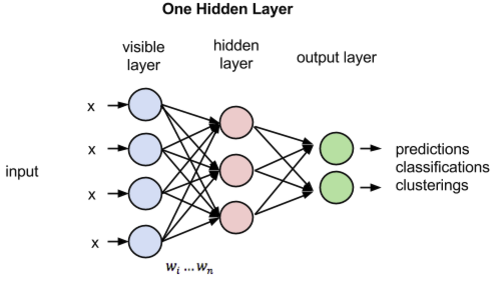隐藏层中的人工神经元，也称单元(units)通常用非线性激励函数，如双曲正切函数(hyperbolic tangent function)和逻辑函数(logistic function)，公式如下所示：
$f(x)=tanh(x)$
$f(x)=1/(1+e^{-x})$
我们的目标是找到成本函数最小化的权重值。通常，MLP的成本函数是残差平方和的均值，计算公式如下所示，其中的 mm 表示训练样本的数量：
2 MLP的训练方法
需要训练的模型参数(parameters)：

num_hidden:隐藏层节点数目
activation func:隐藏层/输出层节点的激发函数
weights/biases:连接权重/偏置

构造成本函数:
$C= 1/2||y -a^L||^2=1/2\sum_{j=0}^n(y_j -a_j^L)^2$
反向传播（backpropagation）算法经常用来连接优化算法求解成本函数最小化问题，比如梯度下降法。这个算法名称是反向（back）和传播（propagation）的合成词，是指误差在网络层的流向。理论上，反向传播可以用于训练具有任意层、任意数量隐藏单元的前馈人工神经网络，但是计算能力的实际限制会约束反向传播的能力。
反向传播与梯度下降法类似，根据成本函数的梯度变化不断更新模型参数。与我们前面介绍过的线性模型不同，神经网络包含不可见的隐藏单元；我们不能从训练集中找到它们。如果我们找不到这些隐藏单元，我们也就不能计算它们的误差，不能计算成本函数的梯度，进而无法求出权重值。如果一个随机变化是某个权重降低了成本函数值，那么我们保留这个变化，就可能同时改变另一个权重的值。这种做法有个明显的问题，就是其计算成本过高。而反向传播算法提供了一种有效的解决方法。
3 MLP的学习
系列文章

深度学习原理与实践(开源图书)-总目录
机器学习原理与实践(开源图书)-总目录
Github: 机器学习&深度学习理论与实践(开源图书)

参考文献

 Ian Goodfellow, Yoshua Bengio. Deep Learning. MIT Press. 2016.
 焦李成等. 深度学习、优化与识别. 清华大学出版社. 2017.
 佩德罗·多明戈斯. 终极算法-机器学习和人工智能如何重塑世界. 中信出版社. 2018.
 雷.库兹韦尔. 人工智能的未来-揭示人类思维的奥秘.  浙江人民出版社. 2016.


展开全文深度学习
• DeepLearning tutorial（3）MLP多层感知机原理简介+代码详解 @author：wepon@blog：http://blog.csdn.net/u012162613/article/details/43221829 本文介绍多层感知机算法，特别是详细解读其代码实现，基于Python ...
>

DeepLearning tutorial（3）MLP多层感知机原理简介+代码详解

@author：wepon

@blog：http://blog.csdn.net/u012162613/article/details/43221829

本文介绍多层感知机算法，特别是详细解读其代码实现，基于Python theano，代码来自：Multilayer
Perceptron，如果你想详细了解多层感知机算法，可以参考：UFLDL教程，或者参考本文第一部分的算法简介。

经详细注释的代码：放在我的github地址上，可下载。

一、多层感知机（MLP）原理简介

多层感知机（MLP，Multilayer Perceptron）也叫人工神经网络（ANN，Artificial Neural Network），除了输入输出层，它中间可以有多个隐层，最简单的MLP只含一个隐层，即三层的结构，如下图：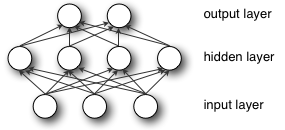从上图可以看到，多层感知机层与层之间是全连接的（全连接的意思就是：上一层的任何一个神经元与下一层的所有神经元都有连接）。多层感知机最底层是输入层，中间是隐藏层，最后是输出层。

输入层没什么好说，你输入什么就是什么，比如输入是一个n维向量，就有n个神经元。

隐藏层的神经元怎么得来？首先它与输入层是全连接的，假设输入层用向量X表示，则隐藏层的输出就是

f(W1X+b1)，W1是权重（也叫连接系数），b1是偏置，函数f 可以是常用的sigmoid函数或者tanh函数：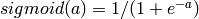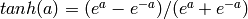最后就是输出层，输出层与隐藏层是什么关系？其实隐藏层到输出层可以看成是一个多类别的逻辑回归，也即softmax回归，所以输出层的输出就是softmax(W2X1+b2)，X1表示隐藏层的输出f(W1X+b1)。

MLP整个模型就是这样子的，上面说的这个三层的MLP用公式总结起来就是，函数G是softmax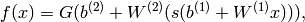因此，MLP所有的参数就是各个层之间的连接权重以及偏置，包括W1、b1、W2、b2。对于一个具体的问题，怎么确定这些参数？求解最佳的参数是一个最优化问题，解决最优化问题，最简单的就是梯度下降法了（SGD）：首先随机初始化所有参数，然后迭代地训练，不断地计算梯度和更新参数，直到满足某个条件为止（比如误差足够小、迭代次数足够多时）。这个过程涉及到代价函数、规则化（Regularization）、学习速率（learning rate）、梯度计算等，本文不详细讨论，读者可以参考本文顶部给出的两个链接。

了解了MLP的基本模型，下面进入代码实现部分。

二、多层感知机（MLP）代码详细解读（基于python+theano）

再次说明，代码来自：Multilayer
Perceptron，本文只是做一个详细解读，如有错误，请不吝指出。

这个代码实现的是一个三层的感知机，但是理解了代码之后，实现n层感知机都不是问题，所以只需理解好这个三层的MLP模型即可。概括地说，MLP的输入层X其实就是我们的训练数据，所以输入层不用实现，剩下的就是“输入层到隐含层”，“隐含层到输出层”这两部分。上面介绍原理时已经说到了，“输入层到隐含层”就是一个全连接的层，在下面的代码中我们把这一部分定义为HiddenLayer。“隐含层到输出层”就是一个分类器softmax回归（也有人叫逻辑回归），在下面的代码中我们把这一部分定义为LogisticRegression。

代码详解开始：

（1）导入必要的python模块

主要是numpy、theano，以及Python自带的os、sys、time模块，这些模块的使用在下面的程序中会看到。

[python] view
plain copy

import os
import sys
import time

import numpy

import theano
import theano.tensor as T（2）定义MLP模型（HiddenLayer+LogisticRegression）

这一部分定义MLP的基本“构件”，即上文一直在提的HiddenLayer和LogisticRegression

HiddenLayer隐含层我们需要定义连接系数W、偏置b，输入、输出，具体的代码以及解读如下：

[python] view
plain copy

class HiddenLayer(object):
def __init__(self, rng, input, n_in, n_out, W=None, b=None,
activation=T.tanh):
”“”
注释：
这是定义隐藏层的类，首先明确：隐藏层的输入即input，输出即隐藏层的神经元个数。输入层与隐藏层是全连接的。
假设输入是n_in维的向量（也可以说时n_in个神经元），隐藏层有n_out个神经元，则因为是全连接，
一共有n_in*n_out个权重，故W大小时(n_in,n_out),n_in行n_out列，每一列对应隐藏层的每一个神经元的连接权重。
b是偏置，隐藏层有n_out个神经元，故b时n_out维向量。
rng即随机数生成器，numpy.random.RandomState，用于初始化W。
input训练模型所用到的所有输入，并不是MLP的输入层，MLP的输入层的神经元个数时n_in，而这里的参数input大小是（n_example,n_in）,每一行一个样本，即每一行作为MLP的输入层。
activation:激活函数,这里定义为函数tanh
”“”

self.input = input   #类HiddenLayer的input即所传递进来的input

”“”
注释：
代码要兼容GPU，则W、b必须使用 dtype=theano.config.floatX,并且定义为theano.shared
另外，W的初始化有个规则：如果使用tanh函数，则在-sqrt(6./(n_in+n_hidden))到sqrt(6./(n_in+n_hidden))之间均匀
抽取数值来初始化W，若时sigmoid函数，则以上再乘4倍。
”“”
#如果W未初始化，则根据上述方法初始化。
#加入这个判断的原因是：有时候我们可以用训练好的参数来初始化W，见我的上一篇文章。
if W is None:
W_values = numpy.asarray(
rng.uniform(
low=-numpy.sqrt(6. / (n_in + n_out)),
high=numpy.sqrt(6. / (n_in + n_out)),
size=(n_in, n_out)
),
dtype=theano.config.floatX
)
if activation == theano.tensor.nnet.sigmoid:
W_values *= 4
W = theano.shared(value=W_values, name=’W’, borrow=True)

if b is None:
b_values = numpy.zeros((n_out,), dtype=theano.config.floatX)
b = theano.shared(value=b_values, name=’b’, borrow=True)

#用上面定义的W、b来初始化类HiddenLayer的W、b
self.W = W
self.b = b

#隐含层的输出
lin_output = T.dot(input, self.W) + self.b
self.output = (
lin_output if activation is None
else activation(lin_output)
)

#隐含层的参数
self.params = [self.W, self.b]LogisticRegression
逻辑回归（softmax回归），代码详解如下。

（如果你想详细了解softmax回归，可以参考： DeepLearning tutorial（1）Softmax回归原理简介+代码详解）

[python] view
plain copy

”“”
定义分类层，Softmax回归
在deeplearning tutorial中，直接将LogisticRegression视为Softmax，
而我们所认识的二类别的逻辑回归就是当n_out=2时的LogisticRegression
”“”
#参数说明：
#input，大小就是(n_example,n_in)，其中n_example是一个batch的大小，
#因为我们训练时用的是Minibatch SGD，因此input这样定义
#n_in,即上一层(隐含层)的输出
#n_out,输出的类别数
class LogisticRegression(object):
def __init__(self, input, n_in, n_out):

#W大小是n_in行n_out列，b为n_out维向量。即：每个输出对应W的一列以及b的一个元素。
self.W = theano.shared(
value=numpy.zeros(
(n_in, n_out),
dtype=theano.config.floatX
),
name=’W’,
borrow=True
)

self.b = theano.shared(
value=numpy.zeros(
(n_out,),
dtype=theano.config.floatX
),
name=’b’,
borrow=True
)

#input是(n_example,n_in)，W是（n_in,n_out）,点乘得到(n_example,n_out)，加上偏置b，
#再作为T.nnet.softmax的输入，得到p_y_given_x
#故p_y_given_x每一行代表每一个样本被估计为各类别的概率
#PS：b是n_out维向量，与(n_example,n_out)矩阵相加，内部其实是先复制n_example个b，
#然后(n_example,n_out)矩阵的每一行都加b
self.p_y_given_x = T.nnet.softmax(T.dot(input, self.W) + self.b)

#argmax返回最大值下标，因为本例数据集是MNIST，下标刚好就是类别。axis=1表示按行操作。
self.y_pred = T.argmax(self.p_y_given_x, axis=1)

#params，LogisticRegression的参数
self.params = [self.W, self.b]ok！这两个基本“构件”做好了，现在我们可以将它们“组装”在一起。

我们要三层的MLP，则只需要HiddenLayer+LogisticRegression，

如果要四层的MLP，则为HiddenLayer+HiddenLayer+LogisticRegression……..以此类推。

下面是三层的MLP：

[python] view
plain copy

#3层的MLP
class MLP(object):
def __init__(self, rng, input, n_in, n_hidden, n_out):

self.hiddenLayer = HiddenLayer(
rng=rng,
input=input,
n_in=n_in,
n_out=n_hidden,
activation=T.tanh
)

#将隐含层hiddenLayer的输出作为分类层logRegressionLayer的输入，这样就把它们连接了
self.logRegressionLayer = LogisticRegression(
input=self.hiddenLayer.output,
n_in=n_hidden,
n_out=n_out
)

#以上已经定义好MLP的基本结构，下面是MLP模型的其他参数或者函数

#规则化项：常见的L1、L2_sqr
self.L1 = (
abs(self.hiddenLayer.W).sum()
+ abs(self.logRegressionLayer.W).sum()
)

self.L2_sqr = (
(self.hiddenLayer.W ** 2).sum()
+ (self.logRegressionLayer.W ** 2).sum()
)

#损失函数Nll（也叫代价函数）
self.negative_log_likelihood = (
self.logRegressionLayer.negative_log_likelihood
)

#误差
self.errors = self.logRegressionLayer.errors

#MLP的参数
self.params = self.hiddenLayer.params + self.logRegressionLayer.params
# end-snippet-3MLP类里面除了隐含层和分类层，还定义了损失函数、规则化项，这是在求解优化算法时用到的。

（3）将MLP应用于MNIST（手写数字识别）

上面定义好了一个三层的MLP，接下来使用它在MNIST数据集上分类，MNIST是一个手写数字0～9的数据集。

[python] view
plain copy

”“”
加载MNIST数据集
”“”
# dataset是数据集的路径，程序首先检测该路径下有没有MNIST数据集，没有的话就下载MNIST数据集
#这一部分就不解释了，与softmax回归算法无关。
data_dir, data_file = os.path.split(dataset)
if data_dir == ”“ and not os.path.isfile(dataset):
# Check if dataset is in the data directory.
new_path = os.path.join(
os.path.split(__file__),
”..”,
”data”,
dataset
)
if os.path.isfile(new_path) or data_file == ‘mnist.pkl.gz’:
dataset = new_path

if (not os.path.isfile(dataset)) and data_file == ‘mnist.pkl.gz’:
import urllib
origin = (
’http://www.iro.umontreal.ca/~lisa/deep/data/mnist/mnist.pkl.gz’
)
urllib.urlretrieve(origin, dataset)

#从”mnist.pkl.gz”里加载train_set, valid_set, test_set，它们都是包括label的
#‘rb’表示以二进制可读的方式打开文件
f = gzip.open(dataset, ’rb’)
f.close()

#将数据设置成shared variables，主要时为了GPU加速，只有shared variables才能存到GPU memory中
#GPU里数据类型只能是float。而data_y是类别，所以最后又转换为int返回
def shared_dataset(data_xy, borrow=True):
data_x, data_y = data_xy
shared_x = theano.shared(numpy.asarray(data_x,
dtype=theano.config.floatX),
borrow=borrow)
shared_y = theano.shared(numpy.asarray(data_y,
dtype=theano.config.floatX),
borrow=borrow)
return shared_x, T.cast(shared_y, ‘int32’)

test_set_x, test_set_y = shared_dataset(test_set)
valid_set_x, valid_set_y = shared_dataset(valid_set)
train_set_x, train_set_y = shared_dataset(train_set)

rval = [(train_set_x, train_set_y), (valid_set_x, valid_set_y),
(test_set_x, test_set_y)]
return rval加载了数据，可以开始训练这个模型了，以下就是主体函数test_mlp()，将MLP用在MNIST上：

[python] view
plain copy

#test_mlp是一个应用实例，用梯度下降来优化MLP，针对MNIST数据集
def test_mlp(learning_rate=0.01, L1_reg=0.00, L2_reg=0.0001, n_epochs=10,
dataset=’mnist.pkl.gz’, batch_size=20, n_hidden=500):
”“”
注释：
learning_rate学习速率，梯度前的系数。
L1_reg、L2_reg：正则化项前的系数，权衡正则化项与Nll项的比重
代价函数=Nll+L1_reg*L1或者L2_reg*L2_sqr
n_epochs：迭代的最大次数（即训练步数），用于结束优化过程
dataset：训练数据的路径
n_hidden:隐藏层神经元个数
batch_size=20，即每训练完20个样本才计算梯度并更新参数
”“”

#加载数据集，并分为训练集、验证集、测试集。
train_set_x, train_set_y = datasets
valid_set_x, valid_set_y = datasets
test_set_x, test_set_y = datasets

#shape获得行数，一行代表一个样本，故获取的是样本数，除以batch_size可以得到有多少个batch
n_train_batches = train_set_x.get_value(borrow=True).shape / batch_size
n_valid_batches = valid_set_x.get_value(borrow=True).shape / batch_size
n_test_batches = test_set_x.get_value(borrow=True).shape / batch_size

######################
# BUILD ACTUAL MODEL #
######################
print ‘… building the model’

#index表示batch的下标，标量
#x表示数据集
#y表示类别，一维向量
index = T.lscalar()
x = T.matrix(’x’)
y = T.ivector(’y’)

rng = numpy.random.RandomState(1234)
#生成一个MLP，命名为classifier
classifier = MLP(
rng=rng,
input=x,
n_in=28 * 28,
n_hidden=n_hidden,
n_out=10
)

#代价函数，有规则化项
#用y来初始化，而其实还有一个隐含的参数x在classifier中
cost = (
classifier.negative_log_likelihood(y)
+ L1_reg * classifier.L1
+ L2_reg * classifier.L2_sqr
)

#这里必须说明一下theano的function函数，givens是字典，其中的x、y是key，冒号后面是它们的value。
#在function被调用时，x、y将被具体地替换为它们的value，而value里的参数index就是inputs=[index]这里给出。
#下面举个例子：
#比如test_model(1)，首先根据index=1具体化x为test_set_x[1 * batch_size: (1 + 1) * batch_size]，
#具体化y为test_set_y[1 * batch_size: (1 + 1) * batch_size]。然后函数计算outputs=classifier.errors(y)，
#这里面有参数y和隐含的x，所以就将givens里面具体化的x、y传递进去。
test_model = theano.function(
inputs=[index],
outputs=classifier.errors(y),
givens={
x: test_set_x[index * batch_size:(index + 1) * batch_size],
y: test_set_y[index * batch_size:(index + 1) * batch_size]
}
)

validate_model = theano.function(
inputs=[index],
outputs=classifier.errors(y),
givens={
x: valid_set_x[index * batch_size:(index + 1) * batch_size],
y: valid_set_y[index * batch_size:(index + 1) * batch_size]
}
)

#cost函数对各个参数的偏导数值，即梯度，存于gparams
gparams = [T.grad(cost, param) for param in classifier.params]

#参数更新规则
#updates[(),(),()….],每个括号里面都是(param, param - learning_rate * gparam)，即每个参数以及它的更新公式
(param, param - learning_rate * gparam)
for param, gparam in zip(classifier.params, gparams)
]

train_model = theano.function(
inputs=[index],
outputs=cost,
givens={
x: train_set_x[index * batch_size: (index + 1) * batch_size],
y: train_set_y[index * batch_size: (index + 1) * batch_size]
}
)

###############
# 开始训练模型 #
###############
print ‘… training’

patience = 10000
patience_increase = 2
#提高的阈值，在验证误差减小到之前的0.995倍时，会更新best_validation_loss
improvement_threshold = 0.995
#这样设置validation_frequency可以保证每一次epoch都会在验证集上测试。
validation_frequency = min(n_train_batches, patience / 2)

best_validation_loss = numpy.inf
best_iter = 0
test_score = 0.
start_time = time.clock()

#epoch即训练步数，每个epoch都会遍历所有训练数据
epoch = 0
done_looping = False

#下面就是训练过程了，while循环控制的时步数epoch，一个epoch会遍历所有的batch，即所有的图片。
#for循环是遍历一个个batch，一次一个batch地训练。for循环体里会用train_model(minibatch_index)去训练模型，
#train_model里面的updatas会更新各个参数。
#for循环里面会累加训练过的batch数iter，当iter是validation_frequency倍数时则会在验证集上测试，
#如果验证集的损失this_validation_loss小于之前最佳的损失best_validation_loss，
#则更新best_validation_loss和best_iter，同时在testset上测试。
#如果验证集的损失this_validation_loss小于best_validation_loss*improvement_threshold时则更新patience。
#当达到最大步数n_epoch时，或者patience<iter时，结束训练
while (epoch < n_epochs) and (not done_looping):
epoch = epoch + 1
for minibatch_index in xrange(n_train_batches):#训练时一个batch一个batch进行的

minibatch_avg_cost = train_model(minibatch_index)
# 已训练过的minibatch数，即迭代次数iter
iter = (epoch - 1) * n_train_batches + minibatch_index
#训练过的minibatch数是validation_frequency倍数，则进行交叉验证
if (iter + 1) % validation_frequency == 0:
# compute zero-one loss on validation set
validation_losses = [validate_model(i) for i
in xrange(n_valid_batches)]
this_validation_loss = numpy.mean(validation_losses)

print(
’epoch %i, minibatch %i/%i, validation error %f %%’ %
(
epoch,
minibatch_index + 1,
n_train_batches,
this_validation_loss * 100.
)
)
#当前验证误差比之前的都小，则更新best_validation_loss，以及对应的best_iter，并且在tsetdata上进行test
if this_validation_loss < best_validation_loss:
if (
this_validation_loss < best_validation_loss *
improvement_threshold
):
patience = max(patience, iter * patience_increase)

best_validation_loss = this_validation_loss
best_iter = iter

test_losses = [test_model(i) for i
in xrange(n_test_batches)]
test_score = numpy.mean(test_losses)

print((‘     epoch %i, minibatch %i/%i, test error of ’
’best model %f %%’) %
(epoch, minibatch_index + 1, n_train_batches,
test_score * 100.))
#patience小于等于iter，则终止训练
if patience <= iter:
done_looping = True
break

end_time = time.clock()
print((‘Optimization complete. Best validation score of %f %% ’
’obtained at iteration %i, with test performance %f %%’) %
(best_validation_loss * 100., best_iter + 1, test_score * 100.))
print >> sys.stderr, (‘The code for file ’ +
os.path.split(__file__) +
’ ran for %.2fm’ % ((end_time - start_time) / 60.))文章完，经详细注释的代码：放在我的github地址上，可下载。

如果有任何错误，或者有说不清楚的地方，欢迎评论留言。


展开全文• 感知机求∂L/∂w： 实现 验证 辅助函数 MLP MLP的向量形式： MLP的损失函数： 感知机求∂L/∂w： 实现 import numpy as np import matplotlib . pyplot as plt class MultiPerceptron ...
•  博客园网站“费弗里”博主的《数据科学学习手札34》 因作者正在进行电力用户短期负荷预测方向学习，正遇到了多层感知机的应用问题，所以搜到博客园中费弗里博主写的的以下资料，进行转载以便学习查阅。...
• 一、简介 　机器学习分为很多个领域，...而我们在机器学习中广泛提及的神经网络学习就是机器学习与神经网络的交叉部分，本篇就将介绍基本的神经元模型、感知机模型的知识以及更进一步的多层感知机的具体应用（注意...
• MLP－多层感知机原理及Matlab实现-附件资源
• 本篇博客是在学习多层感知机原理的基础上，借助pytorch深度学习框架运行的一个简单的多层感知机模型，有助于自己对多层感知机模型原理的理解，以及熟练对pytorch工具的应用神经网络 机器学习 python 深度学习 可视化
• 1、多层感知机原理MLP | DNN 前向传播 后向传播 激活函数 梯度下降 2、代码实现对鸢尾花数据分类 主程序：分类结果最终可到达1.0，完全正确 import tensorflow as tf from data_iris import train_...
• 一、多层感知机（MLP）原理简介 多层感知机（MLP，Multilayer Perceptron）也叫人工神经网络（ANN，Artificial Neural Network），除了输入输出层，它中间可以有多个隐层，最简单的MLP只含一个隐层，即三层的结构，...
• 一、多层感知机（MLP）原理简介多层感知机（MLP，Multilayer Perceptron）也叫人工神经网络（ANN，Artificial Neural Network），除了输入输出层，它中间可以有多个隐层，最简单的MLP只含一个隐层，即三层的结构，如...
• ## MLP－多层感知机的原理及Matlab实现

千次阅读 热门讨论 2017-07-02 19:47:46
在此记录一下多层感知机模型的原理以及我自己的感悟。如果有任何疑问欢迎大家跟我讨论：shitianqi1994@163.com感知机matlab实现关于这个看过很多资料，很多资料好像还将感知机和人体大脑感知系统联系起来什么的，...matlab 调试
• ## 多层感知机（MLP）简介

万次阅读 多人点赞 2019-06-23 21:36:59
一、多层感知机（MLP）原理简介 多层感知机（MLP，Multilayer Perceptron）也叫人工神经网络（ANN，Artificial Neural Network），除了输入输出层，它中间可以有多个隐层，最简单的MLP只含一个隐层，即三层的结构...
• 一、多层感知机（MLP）原理简介 多层感知机（MLP，Multilayer Perceptron）也叫人工神经网络（ANN，Artificial Neural Network），除了输入输出层，它中间可以有多个隐层，最简单的MLP只含一个隐层，即三层的结构，...
• 多层感知机matlab代码实现，分类是采用较难分类的半月形两类问题分类，可方便深度学习初学者了解深度学习反馈传播机制。...